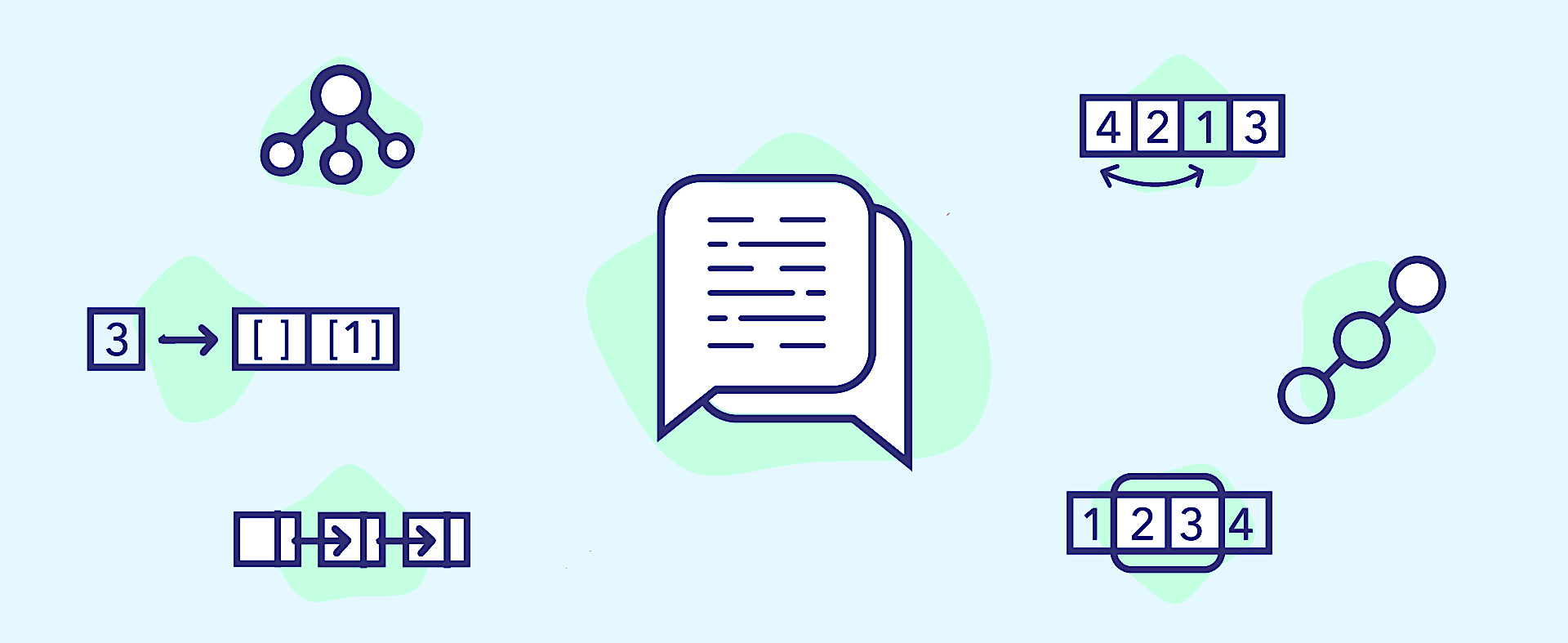Related Tags

java
communitycreator

# How to solve the string rotation problem in Java with a trickJavin Paul

### Problem statement

One of the interesting string algorithm-based coding questions is how do you find if a given string is the rotation of another given string? For example, if the two given strings are “Java” and “aJav,” then they are rotations of each other because it seems the string is rotated from the last “a.” However, “Java” and “Jaav” are not rotations of each other.

You need to write a function in Java that accepts two string inputs and returns true if they are rotations of each other and false otherwise. This question might seem difficult, but there is a nice trick to solve this problem, which we’ll go over in this shot.

### Things to consider

Like all other programming interview questions, you should ask some general questions before jumping into the solution, e.g., are the given strings in ASCII or Unicode? Since ASCII and Unicode are different encoding schemes, it will affect the solution. For this question, we’ll assume that the given strings are in ASCII format.

Another good question to ask while dealing with string interview questions is about case sensitivity. Does your solution need to consider the case or not?

For example, if the case is not considered, then ‘a’ and ‘A’ will be treated equally. Hence, ‘java’ and ‘aJav’ will be rotations of each other.

Here, we can assume the solution is case-sensitive.

Similarly, you can ask whether you can use additional data structures or not, how big the string will be, whether both strings can fit in memory or not, etc.

These questions show your attention to detail, which is very valuable when working with real-life production systems.

### Solution

Checking if a string is the rotation of the other has quite a lot of similarity with the permutation of a string, e.g., both the original and rotation of the string have the same length.

Moreover, in rotation, characters are rotated around other characters (a pivot), so when you concatenate the original string with itself and take the substring with the rotated string, you can always find the substring in the concatenation.

### Algorithm

The algorithm to check if one string is the rotation of the other is as follows:

1. Concatenate the original string with itself.

2. Check if the second string exists in the concatenated string. If yes, then the second string is the rotation of the first string; otherwise, it’s not.

Let’s look at an example to see this algorithm in action.

Suppose the given strings are “abcd” and “dabc.”

1. Concatenate “abcd” + “abcd” so that it becomes “abcdabcd.”

2. Use the indexOf method to check if “dbac” exists in “abcdabcd” or not. Since it does, “dabc” is a valid rotation of the string “abcd.”

### Code

public class main{

public static void main(String args[]){

System.out.println("Is army rotation of myar");
if(isRotation("army", "myar")){
System.out.println("yes");
}else{
System.out.println("no");
}

}

public static boolean isRotation(String first, String second) {
if (first.length() != second.length()) {
return false;
}

String concatenated = first + first;

if (concatenated.indexOf(second) != -1) {
return true;
}

return false;
}
}

### Explanation

There can only be N rotations of a string if it contains N characters, and when you join the string with itself, you get all the rotations in one string.

The time and space complexity of this solution is $O(n)$.

This is one of the easier coding problems you can get during Java interviews, but nowadays, it’s getting tougher and tougher, and you may be asked to solve dynamic programming-based problems.

I suggest becoming familiar with essential coding patterns like sliding window, merge interval, two pointers approach, and top k elements.

If you need a resource, I highly recommend Grokking the Coding Interview: Patterns for Coding Questions.If you have any questions, you can always reach out to me. I share my thoughts on javarevisited and java67.com.

RELATED TAGS

java
communitycreator

CONTRIBUTORJavin Paul
RELATED COURSES

View all Courses

Keep Exploring

Learn in-demand tech skills in half the time